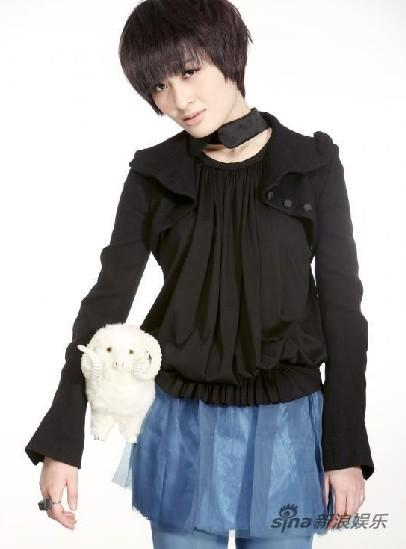# 12星座的代表歌曲-看看你的是什么

## 12星座的代表歌曲-看看你的是什么

I remember remember 谁说过爱情 必须要望穿秋水刻骨铭心
Sorry so sorry 我只会忽然之间轻轻想起你
Sorry sorry 只是忽然很想你
Sorry sorry 这样算不算爱情==--==--==--==--==--==--==--==--==--==--==--==--==--==--==--==--==--==--==--==--==--==--==--==--==--==--==--==--==--==--==--==--==--==--==--==--==--==--==--==--==--==--==--==--==--==--==--==--==--==--==--==--==--==--==--==--==--==--==--==--==--==--==--==--==--==--==--==--==--==--==--==--==--==--==--==--==--==--==--==--==--==--==--==--==--==--==--==--==--==--

oh baby

baby我已不能爱你多一些==--==--==--==--==--==--==--==--==--==--==--==--==--==--==--==--==--==--==--==--==--==--==--==--==--==--==--==--==--==--==--==--==--==--==--==--==--==--==--==--==--==--==--==--==--==--==--==--==--==--==--==--==--==--==--==--==--==--==--==--==--==--==--==--==--==--==--==--==--==--==--==--

i give you face
you don't wanna face
i true my face==--==--==--==--==--==--==--==--==--==--==--==--==--==--==--==--==--==--==--==--==--==--==--==--==--==--==--==--==--==--==--==--==--==--==--==--TOP

Processed in 0.008551 second(s), 6 queries, Gzip enabled.Powered by Discuz! 6.0.0 © 2001-2007 Comsenz Inc. 上外论坛,上外bbs